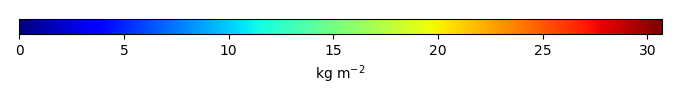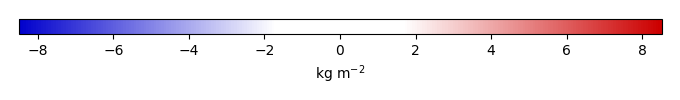# Mean State

Period Mean (original grids) [Pg]
Model Period Mean (intersection) [Pg]
Model Period Mean (complement) [Pg]
Benchmark Period Mean (intersection) [Pg]
Benchmark Period Mean (complement) [Pg]
Bias [kg m-2]
Bias Score 
Spatial Distribution Score 
Overall Score 
Benchmark [-] 32.8
CLM4 [-] 49.5 50.3 0.182 32.8 0.0526 4.47 0.573 0.760 0.667
CLM4.5 [-] 45.4 45.4 0.235 32.8 0.0526 3.37 0.538 0.714 0.626
CLM5 [-] 38.5 38.7 0.241 32.8 0.0526 1.73 0.717 0.926 0.822
Period Mean (original grids) [Pg]
Model Period Mean (intersection) [Pg]
Model Period Mean (complement) [Pg]
Benchmark Period Mean (intersection) [Pg]
Benchmark Period Mean (complement) [Pg]
Bias [kg m-2]
Bias Score 
Spatial Distribution Score 
Overall Score 
Benchmark [-] 8.35
CLM4 [-] 7.61 6.74 0.0800 8.34 0.0114 -0.268 0.634 0.910 0.772
CLM4.5 [-] 2.26 1.89 0.152 8.34 0.0114 -1.12 0.444 0.398 0.421
CLM5 [-] 3.24 2.69 0.201 8.34 0.0114 -0.977 0.477 0.622 0.550
Period Mean (original grids) [Pg]
Model Period Mean (intersection) [Pg]
Model Period Mean (complement) [Pg]
Benchmark Period Mean (intersection) [Pg]
Benchmark Period Mean (complement) [Pg]
Bias [kg m-2]
Bias Score 
Spatial Distribution Score 
Overall Score 
Benchmark [-] 10.6
CLM4 [-] 10.9 10.4 0.214 10.6 0.0246 0.0746 0.549 0.640 0.595
CLM4.5 [-] 4.95 4.63 0.213 10.6 0.0246 -0.565 0.457 0.690 0.573
CLM5 [-] 12.3 11.7 0.363 10.6 0.0246 0.299 0.500 0.561 0.530
Period Mean (original grids) [Pg]
Model Period Mean (intersection) [Pg]
Model Period Mean (complement) [Pg]
Benchmark Period Mean (intersection) [Pg]
Benchmark Period Mean (complement) [Pg]
Bias [kg m-2]
Bias Score 
Spatial Distribution Score 
Overall Score 
Benchmark [-] 4.69
CLM4 [-] 0.798 0.806 0.0186 4.67 0.0241 -1.12 0.441 0.260 0.351
CLM4.5 [-] 3.11 3.09 0.0372 4.67 0.0241 -0.401 0.608 0.675 0.641
CLM5 [-] 4.17 4.12 0.0636 4.67 0.0241 -0.0436 0.581 0.798 0.689
Period Mean (original grids) [Pg]
Model Period Mean (intersection) [Pg]
Model Period Mean (complement) [Pg]
Benchmark Period Mean (intersection) [Pg]
Benchmark Period Mean (complement) [Pg]
Bias [kg m-2]
Bias Score 
Spatial Distribution Score 
Overall Score 
Benchmark [-] 27.8
CLM4 [-] 62.8 60.6 1.99 26.8 0.921 15.4 0.313 0.631 0.472
CLM4.5 [-] 54.1 52.1 1.81 26.8 0.921 13.0 0.392 0.824 0.608
CLM5 [-] 39.9 38.2 1.51 26.8 0.921 7.87 0.607 0.919 0.763
Period Mean (original grids) [Pg]
Model Period Mean (intersection) [Pg]
Model Period Mean (complement) [Pg]
Benchmark Period Mean (intersection) [Pg]
Benchmark Period Mean (complement) [Pg]
Bias [kg m-2]
Bias Score 
Spatial Distribution Score 
Overall Score 
Benchmark [-] 43.5
CLM4 [-] 26.8 26.1 0.0959 43.5 0.0288 -1.84 0.586 0.860 0.723
CLM4.5 [-] 31.7 31.0 0.174 43.5 0.0288 -1.27 0.588 0.855 0.721
CLM5 [-] 52.5 51.4 0.326 43.5 0.0288 1.03 0.493 0.626 0.560
Period Mean (original grids) [Pg]
Model Period Mean (intersection) [Pg]
Model Period Mean (complement) [Pg]
Benchmark Period Mean (intersection) [Pg]
Benchmark Period Mean (complement) [Pg]
Bias [kg m-2]
Bias Score 
Spatial Distribution Score 
Overall Score 
Benchmark [-] 32.0
CLM4 [-] 40.6 40.6 0.605 31.9 0.0804 0.794 0.564 0.774 0.669
CLM4.5 [-] 40.9 40.7 0.650 31.9 0.0804 0.833 0.516 0.737 0.626
CLM5 [-] 31.6 31.8 0.632 31.9 0.0804 0.262 0.606 0.861 0.734
Period Mean (original grids) [Pg]
Model Period Mean (intersection) [Pg]
Model Period Mean (complement) [Pg]
Benchmark Period Mean (intersection) [Pg]
Benchmark Period Mean (complement) [Pg]
Bias [kg m-2]
Bias Score 
Spatial Distribution Score 
Overall Score 
Benchmark [-] 21.1
CLM4 [-] 28.1 26.8 0.449 20.9 0.146 1.69 0.562 0.731 0.646
CLM4.5 [-] 22.9 21.6 0.529 20.9 0.146 1.10 0.491 0.656 0.574
CLM5 [-] 24.8 23.3 0.610 20.9 0.146 1.28 0.600 0.820 0.710
Period Mean (original grids) [Pg]
Model Period Mean (intersection) [Pg]
Model Period Mean (complement) [Pg]
Benchmark Period Mean (intersection) [Pg]
Benchmark Period Mean (complement) [Pg]
Bias [kg m-2]
Bias Score 
Spatial Distribution Score 
Overall Score 
Benchmark [-] 3.81
CLM4 [-] 0.743 0.815 0.0110 3.81 0.000319 -0.856 0.453 0.288 0.370
CLM4.5 [-] 3.24 3.34 0.0509 3.81 0.000319 -0.123 0.629 0.699 0.664
CLM5 [-] 6.88 7.17 0.0941 3.81 0.000319 1.03 0.512 0.754 0.633
Period Mean (original grids) [Pg]
Model Period Mean (intersection) [Pg]
Model Period Mean (complement) [Pg]
Benchmark Period Mean (intersection) [Pg]
Benchmark Period Mean (complement) [Pg]
Bias [kg m-2]
Bias Score 
Spatial Distribution Score 
Overall Score 
Benchmark [-] 9.16
CLM4 [-] 7.63 7.09 0.450 8.87 0.289 0.206 0.570 0.825 0.697
CLM4.5 [-] 7.65 7.05 0.527 8.87 0.289 0.239 0.580 0.838 0.709
CLM5 [-] 11.0 10.0 0.829 8.87 0.289 0.760 0.605 0.812 0.708
Period Mean (original grids) [Pg]
Model Period Mean (intersection) [Pg]
Model Period Mean (complement) [Pg]
Benchmark Period Mean (intersection) [Pg]
Benchmark Period Mean (complement) [Pg]
Bias [kg m-2]
Bias Score 
Spatial Distribution Score 
Overall Score 
Benchmark [-] 12.0
CLM4 [-] 5.40 5.21 0.116 12.0 0.0529 -0.977 0.535 0.665 0.600
CLM4.5 [-] 11.2 10.8 0.257 12.0 0.0529 0.212 0.629 0.849 0.739
CLM5 [-] 18.2 17.4 0.537 12.0 0.0529 1.77 0.466 0.349 0.407
Period Mean (original grids) [Pg]
Model Period Mean (intersection) [Pg]
Model Period Mean (complement) [Pg]
Benchmark Period Mean (intersection) [Pg]
Benchmark Period Mean (complement) [Pg]
Bias [kg m-2]
Bias Score 
Spatial Distribution Score 
Overall Score 
Benchmark [-] 368.
CLM4 [-] 467. 460. 6.82 363. 4.64 1.23 0.521 0.637 0.579
CLM4.5 [-] 453. 445. 8.16 363. 4.64 1.17 0.530 0.711 0.621
CLM5 [-] 487. 477. 9.77 363. 4.64 1.46 0.573 0.784 0.679
Period Mean (original grids) [Pg]
Model Period Mean (intersection) [Pg]
Model Period Mean (complement) [Pg]
Benchmark Period Mean (intersection) [Pg]
Benchmark Period Mean (complement) [Pg]
Bias [kg m-2]
Bias Score 
Spatial Distribution Score 
Overall Score 
Benchmark [-] 1.24
CLM4 [-] 1.32 1.18 0.143 1.23 0.00783 0.0265 0.555 0.774 0.665
CLM4.5 [-] 1.34 1.15 0.206 1.23 0.00783 0.0355 0.519 0.651 0.585
CLM5 [-] 1.55 1.22 0.341 1.23 0.00783 0.0659 0.507 0.738 0.623
Period Mean (original grids) [Pg]
Model Period Mean (intersection) [Pg]
Model Period Mean (complement) [Pg]
Benchmark Period Mean (intersection) [Pg]
Benchmark Period Mean (complement) [Pg]
Bias [kg m-2]
Bias Score 
Spatial Distribution Score 
Overall Score 
Benchmark [-] 5.62
CLM4 [-] 3.07 3.02 0.0260 5.59 0.0265 -0.391 0.590 0.695 0.643
CLM4.5 [-] 7.93 7.70 0.111 5.59 0.0265 0.446 0.602 0.865 0.733
CLM5 [-] 14.5 14.1 0.228 5.59 0.0265 1.60 0.345 0.405 0.375
Period Mean (original grids) [Pg]
Model Period Mean (intersection) [Pg]
Model Period Mean (complement) [Pg]
Benchmark Period Mean (intersection) [Pg]
Benchmark Period Mean (complement) [Pg]
Bias [kg m-2]
Bias Score 
Spatial Distribution Score 
Overall Score 
Benchmark [-] 8.21
CLM4 [-] 4.65 4.16 0.219 8.09 0.122 -0.578 0.464 0.538 0.501
CLM4.5 [-] 8.49 7.87 0.366 8.09 0.122 0.956 0.483 0.505 0.494
CLM5 [-] 10.2 9.46 0.474 8.09 0.122 1.74 0.539 0.599 0.569
Period Mean (original grids) [Pg]
Model Period Mean (intersection) [Pg]
Model Period Mean (complement) [Pg]
Benchmark Period Mean (intersection) [Pg]
Benchmark Period Mean (complement) [Pg]
Bias [kg m-2]
Bias Score 
Spatial Distribution Score 
Overall Score 
Benchmark [-] 14.8
CLM4 [-] 13.4 13.1 0.144 14.8 0.0147 -0.146 0.691 0.906 0.798
CLM4.5 [-] 14.7 14.3 0.191 14.8 0.0147 0.212 0.631 0.782 0.707
CLM5 [-] 12.0 11.7 0.182 14.8 0.0147 -0.425 0.652 0.870 0.761
Period Mean (original grids) [Pg]
Model Period Mean (intersection) [Pg]
Model Period Mean (complement) [Pg]
Benchmark Period Mean (intersection) [Pg]
Benchmark Period Mean (complement) [Pg]
Bias [kg m-2]
Bias Score 
Spatial Distribution Score 
Overall Score 
Benchmark [-] 20.1
CLM4 [-] 16.3 16.3 0.135 19.8 0.219 -0.452 0.595 0.782 0.688
CLM4.5 [-] 28.1 28.1 0.187 19.8 0.219 1.88 0.527 0.862 0.695
CLM5 [-] 43.6 43.6 0.247 19.8 0.219 4.91 0.403 0.721 0.562
Period Mean (original grids) [Pg]
Model Period Mean (intersection) [Pg]
Model Period Mean (complement) [Pg]
Benchmark Period Mean (intersection) [Pg]
Benchmark Period Mean (complement) [Pg]
Bias [kg m-2]
Bias Score 
Spatial Distribution Score 
Overall Score 
Benchmark [-] 8.04
CLM4 [-] 4.00 4.04 0.0137 7.99 0.0515 -0.912 0.516 0.275 0.396
CLM4.5 [-] 2.99 3.01 0.0287 7.99 0.0515 -1.13 0.487 0.259 0.373
CLM5 [-] 3.04 3.04 0.0352 7.99 0.0515 -1.10 0.511 0.490 0.500
Period Mean (original grids) [Pg]
Model Period Mean (intersection) [Pg]
Model Period Mean (complement) [Pg]
Benchmark Period Mean (intersection) [Pg]
Benchmark Period Mean (complement) [Pg]
Bias [kg m-2]
Bias Score 
Spatial Distribution Score 
Overall Score 
Benchmark [-] 88.9
CLM4 [-] 159. 158. 0.324 88.8 0.0655 8.54 0.467 0.552 0.510
CLM4.5 [-] 141. 141. 0.389 88.8 0.0655 6.54 0.547 0.736 0.641
CLM5 [-] 129. 128. 0.373 88.8 0.0655 5.00 0.646 0.945 0.795
Period Mean (original grids) [Pg]
Model Period Mean (intersection) [Pg]
Model Period Mean (complement) [Pg]
Benchmark Period Mean (intersection) [Pg]
Benchmark Period Mean (complement) [Pg]
Bias [kg m-2]
Bias Score 
Spatial Distribution Score 
Overall Score 
Benchmark [-] 9.09
CLM4 [-] 17.8 17.9 0.101 9.08 0.0128 1.48 0.405 0.245 0.325
CLM4.5 [-] 13.6 13.5 0.162 9.08 0.0128 0.827 0.473 0.282 0.377
CLM5 [-] 21.8 21.8 0.283 9.08 0.0128 2.13 0.323 0.298 0.311
Period Mean (original grids) [Pg]
Model Period Mean (intersection) [Pg]
Model Period Mean (complement) [Pg]
Benchmark Period Mean (intersection) [Pg]
Benchmark Period Mean (complement) [Pg]
Bias [kg m-2]
Bias Score 
Spatial Distribution Score 
Overall Score 
Benchmark [-] 2.45
CLM4 [-] 4.80 5.05 0.0796 2.40 0.0496 1.35 0.392 0.274 0.333
CLM4.5 [-] 5.01 5.08 0.102 2.40 0.0496 1.50 0.350 0.281 0.316
CLM5 [-] 6.26 6.15 0.140 2.40 0.0496 1.92 0.352 0.318 0.335

# Temporally integrated period mean

BENCHMARK MEANMODEL MEANBIASBIAS SCORESPATIAL TAYLOR DIAGRAMMODEL COLORS# Spatially integrated regional mean

MODEL COLORS# All Models

BenchmarkCLM4CLM4.5CLM5# Data Information

Approach: To convert the original data in0.25x0.25 to the final one at 0.5x0.5 degree resolution, Please see conversion steps listed in http://redwood.ess.uci.edu/mingquan/www/ILAMB/Download/CODES/CODES/subroutines/convert/readme_biomass. 3 codes below will be used in these steps. Code 1: http://redwood.ess.uci.edu/mingquan/www/ILAMB/Download/CODES/CODES/subroutines/convert/read-GlobalCarbon.pro Code 2: http://redwood.ess.uci.edu/mingquan/www/ILAMB/Download/CODES/CODES/subroutines/convert/convert-GlobalCarbon.ncl Code 3: http://redwood.ess.uci.edu/mingquan/www/ILAMB/Download/CODES/CODES/subroutines/convert/write-GlobalCarbon.ncl First, I used Code 1 to read above ground and below ground biomass carbon data in quater degree from the original geotiff file; then I used Code 2 to convert unit from the orignal unit of ton C per hectare to the final one of Kg C per m2, meanwhile I summed all available data points for both above and below ground data in each 0.5x0.5 grid cell to obtain the total biomass in 0.5x0.5 degree resolution; finally I used Code 3 to create the final file in netCDF format.

Temporal resolution: climatology

General information: This product was derived from global biomass carbon stocks data originally in 0.25x0.25 degree resolution.

Spatial resolution: 0.5x0.5 degree